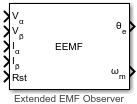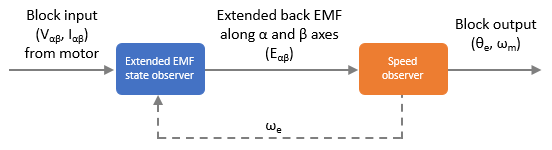# Extended EMF Observer

Compute electrical position and mechanical speed of permanent magnet synchronous motor (PMSM)

Since R2023a

•Libraries:
Motor Control Blockset / Sensorless Estimators

## Description

The Extended EMF Observer block computes the electrical position, θe (or sin θe and cos θe), in addition to the mechanical speed of the PMSM from the measured voltage and current in the stationary αβ reference frame.

The block includes a linear extended EMF state observer that estimates the extended back-EMF. It uses a lowpass filter to remove any noise in the estimated back-EMF value. In addition, the block includes a speed feedforward-based quadrature phase-locked loop (PLL) that acts as a speed observer. The speed observer uses the computed extended back-EMF to determine the motor speed. It calculates speed with zero error even during constant angular acceleration. The block then computes the position output from the estimated motor electrical speed.### Equations

The IPMSM state equation is defined as:

`$\begin{array}{l}\left[\begin{array}{c}\stackrel{·}{i}\\ \stackrel{·}{e}\end{array}\right]=\left[\begin{array}{cc}{A}_{11}& {A}_{12}\\ 0& {A}_{22}\end{array}\right]\left[\begin{array}{c}i\\ e\end{array}\right]+\left[\begin{array}{c}{B}_{1}\\ 0\end{array}\right]v+\left[\begin{array}{c}0\\ W\end{array}\right]\\ i=C\left[\begin{array}{c}i\\ e\end{array}\right]\end{array}$`

These equations describe the reduced order PMSM state model:

`$\begin{array}{l}\stackrel{·}{i}={A}_{11}i+{A}_{12}e+{B}_{1}v\\ \stackrel{·}{e}={A}_{22}e\end{array}$`

Extended back-EMF, $e=\left\{\left({L}_{d}-{L}_{q}\right)\left({\omega }_{re}{i}_{d}-{\stackrel{·}{i}}_{q}\right)+{\omega }_{re}{K}_{E}\right\}\left[\begin{array}{c}-\mathrm{sin}{\theta }_{re}\\ \mathrm{cos}{\theta }_{re}\end{array}\right]$

This equation describes the reduced dimensional state observer:

`$\stackrel{·}{\stackrel{^}{e}}=\left({\stackrel{^}{A}}_{22}-\xi {A}_{12}\right)\stackrel{^}{e}+\xi \left(\stackrel{·}{i}-{\stackrel{^}{A}}_{11}i-{B}_{1}v\right)$`

These equations describe the extended EMF observer parameters:

`$\begin{array}{l}{A}_{22}-\xi {A}_{12}={\omega }_{re}J-\left(\alpha I+\beta J\right)\left(\frac{-1}{{L}_{d}}\right)I\\ \xi =\left(\alpha I+\beta J\right)\end{array}$`

To ensure convergence, if you set $\beta ={\omega }_{re}×{L}_{d}$ and set α to a negative value, then the extended EMF observer gain can be determined as:

where:

• $i=\left[\begin{array}{c}{i}_{\alpha }\\ {i}_{\beta }\end{array}\right]$, $e=\left[\begin{array}{c}{e}_{\alpha }\\ {e}_{\beta }\end{array}\right]$, and $v=\left[\begin{array}{c}{v}_{\alpha }\\ {v}_{\beta }\end{array}\right]$.

• $W=\left({L}_{d}-{L}_{q}\right)\left({\omega }_{re}\stackrel{·}{{i}_{d}}-\stackrel{··}{{i}_{q}}\right)\left[\begin{array}{c}-\mathrm{sin}{\theta }_{re}\\ \mathrm{cos}{\theta }_{re}\end{array}\right]$

• $A=\left[\begin{array}{cc}{A}_{11}& {A}_{12}\\ 0& {A}_{22}\end{array}\right]$, $B=\left[\begin{array}{c}{B}_{1}\\ 0\end{array}\right]$, and $C=\left[\begin{array}{cc}I& 0\end{array}\right]$.

• ${A}_{11}=-\left(\frac{R}{{L}_{d}}\right)I+\left({\omega }_{re}\frac{\left({L}_{d}-{L}_{q}\right)}{{L}_{d}}\right)J$, ${A}_{12}=\left(\frac{-1}{{L}_{d}}\right)I$, and ${A}_{22}={\omega }_{re}J$.

• ${B}_{1}=\left(\frac{1}{{L}_{d}}\right)I$, $I=\left[\begin{array}{cc}1& 0\\ 0& 1\end{array}\right]$, and $J=\left[\begin{array}{cc}0& -1\\ 1& 0\end{array}\right]$.

Note

A and C are an observable pair.

• "$\stackrel{·}{}$" and "$\stackrel{·}{}$$\stackrel{·}{}$" are the first-order and second-order differential operators, respectively.

• vα and vβ are the stator voltages along the α- and β-axes, respectively.

• iα and iβ are the stator currents along the α- and β-axes, respectively.

• eα and eβ are the extended back-EMF along the α- and β-axes, respectively.

• id and iq are the stator currents along the d- and q-axes, respectively.

• R is the stator resistance of PMSM.

• Ld and Lq are the stator inductances of PMSM across d and q axes, respectively.

• KE is the EMF constant

• ωre is the angular velocity at electrical angle.

• θre is the rotor position at electrical angle.

• are the estimated values.

• ξ is the feedback gain.

## Ports

### Input

expand all

Per-unit voltage component along the α-axis of the stationary αβ reference frame.

Data Types: `single` | `double` | `fixed point`

Per-unit voltage component along the β-axis of the stationary αβ reference frame.

Data Types: `single` | `double` | `fixed point`

Per-unit current component along the α-axis of the stationary αβ reference frame.

Data Types: `single` | `double` | `fixed point`

Per-unit current component along the β-axis of the stationary αβ reference frame.

Data Types: `single` | `double` | `fixed point`

The pulse (true value) that resets and restarts the processing of the block algorithm.

Data Types: `single` | `double` | `fixed point`

### Output

expand all

The estimated electrical position of the rotor.

#### Dependencies

To enable this port, set the Position output parameter to `Electrical position`.

Data Types: `single` | `double` | `fixed point`

Sine of the estimated electrical position of the rotor.

#### Dependencies

To enable this port, set the Position output parameter to `Sine and Cosine electrical position`.

Data Types: `single` | `double` | `fixed point`

Cosine of the estimated electrical position of the rotor.

#### Dependencies

To enable this port, set the Position output parameter to `Sine and Cosine electrical position`.

Data Types: `single` | `double` | `fixed point`

The estimated mechanical speed of the rotor.

Data Types: `single` | `double` | `fixed point`

## Parameters

expand all

Units of inputs to the block.

Type of positions that the block outputs.

• `Electrical position` — Select this value to enable the `θe` port.

• `Sine and Cosine electrical position` — Select this value to enable the `Sin θe` and `Cos θe` ports.

The fixed time interval (in seconds) between two consecutive instances of block execution.

### Motor Parameters

Stator phase winding resistance (in ohm).

Stator phase winding inductance (in Henry) along the d-axis of the rotating d-q reference frame.

Stator phase winding inductance (in Henry) along the q-axis of the rotating d-q reference frame.

Maximum mechanical speed (in RPM) that the block can support. If you set the speed to a value higher than this value, the block generates incorrect outputs.

Note

The block uses this parameter to filter out noise in the estimated speed signal.

Number of pole pairs available in the motor.

The maximum phase voltage applied to the PMSM. For details, see Per-Unit System.

The maximum measurable current supplied to the PMSM. For details, see Per-Unit System.

### Observer Parameters

Gain of the linear extended EMF state observer.

Cutoff frequency of the lowpass filter that the block uses to remove noise in the estimated back-EMF value.

Proportional gain of the speed observer used by the block.

Integral gain of the speed observer used by the block.

Number of data points in the lookup table that the speed observer uses to compute speed from the extended back-EMF value.

Cutoff frequency of the lowpass filter that the block uses to remove noise in the estimated speed.

### Datatypes

Unit of the position output.

Data type of the position output.

Unit of the speed output.

Data type of the speed output.

 Zhiqian Chen, M. Tomita, S. Doki and S. Okuma, "An extended electromotive force model for sensorless control of interior permanent-magnet synchronous motors," in IEEE Transactions on Industrial Electronics, vol. 50, no. 2, pp. 288-295, April 2003, doi: 10.1109/TIE.2003.809391.

 T. Ohnuma, S. Doki and S. Okuma, "Extended EMF observer for wide speed range sensorless control of salient-pole synchronous motor drives," The XIX International Conference on Electrical Machines - ICEM 2010, Rome, Italy, 2010, pp. 1-6, doi: 10.1109/ICELMACH.2010.5608087.

 G. Liu, H. Zhang and X. Song, "Position-Estimation Deviation-Suppression Technology of PMSM Combining Phase Self-Compensation SMO and Feed-Forward PLL," in IEEE Journal of Emerging and Selected Topics in Power Electronics, vol. 9, no. 1, pp. 335-344, Feb. 2021, doi: 10.1109/JESTPE.2020.2967508.